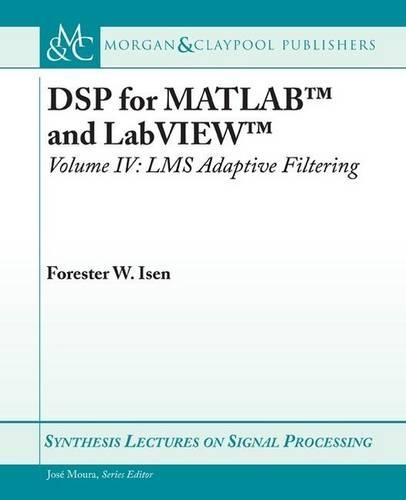•DSP for MATLAB ™ and LabVIEW ™ IV: LMS Adaptive Filters - Synthesis Lectures on Signal Processing (Paperback)

(author)
£41.95
Paperback 127 Pages / Published: 30/01/2009
• We can order this

Usually dispatched within 3 weeks

This book is Volume IV of the series DSP for MATLAB ™ and LabVIEW ™. Volume IV is an introductory treatment of LMS Adaptive Filtering and applications, and covers cost functions, performance surfaces, coefficient perturbation to estimate the gradient, the LMS algorithm, response of the LMS algorithm to narrow-band signals, and various topologies such as ANC (Active Noise Cancelling) or system modeling, Noise Cancellation, Interference Cancellation, Echo Cancellation (with single- and dual-H topologies), and Inverse Filtering/Deconvolution. The entire series consists of four volumes that collectively cover basic digital signal processing in a practical and accessible manner, but which nonetheless include all essential foundation mathematics. As the series title implies, the scripts here will run on both MATLAB ™ and LabVIEW ™. The text for all volumes contains many examples, and many useful computational scripts, augmented by demonstration scripts and LabVIEW ™ Virtual Instruments (VIs) that can be run to illustrate various signal processing concepts graphically on the user's computer screen. Volume I consists of four chapters that collectively set forth a brief overview of the field of digital signal processing, useful signals and concepts (including convolution, recursion, difference equations, LTI systems, etc), conversion from the continuous to discrete domain and back (i.e., analog-to-digital and digital-to-analog conversion), aliasing, the Nyquist rate, normalized frequency, sample rate conversion and Mu-law compression, and signal processing principles including correlation, the correlation sequence, the Real DFT, correlation by convolution, matched filtering, simple FIR filters, and simple IIR filters. Chapter 4 of Volume I, in particular, provides an intuitive or ""first principle"" understanding of how digital filtering and frequency transforms work. Volume II provides detailed coverage of discrete frequency transforms, including a brief overview of common frequency transforms, both discrete and continuous, followed by detailed treatments of the Discrete Time Fourier Transform (DTFT), the z-Transform (including definition and properties, the inverse z-transform, frequency response via z-transform, and alternate filter realization topologies including Direct Form, Direct Form Transposed, Cascade Form, Parallel Form, and Lattice Form), and the Discrete Fourier Transform (DFT) (including Discrete Fourier Series, the DFT-IDFT pair, DFT of common signals, bin width, sampling duration, and sample rate, the FFT, the Goertzel Algorithm, Linear, Periodic, and Circular convolution, DFT Leakage, and computation of the Inverse DFT). Volume III covers digital filter design, including the specific topics of FIR design via windowed-ideal-lowpass filter, FIR highpass, bandpass, and bandstop filter design from windowed-ideal lowpass filters, FIR design using the transition-band-optimized Frequency Sampling technique (implemented by Inverse-DFT or Cosine/Sine Summation Formulas), design of equiripple FIRs of all standard types including Hilbert Transformers and Differentiators via the Remez Exchange Algorithm, design of Butterworth, Chebyshev (Types I and II), and Elliptic analog prototype lowpass filters, conversion of analog lowpass prototype filters to highpass, bandpass, and bandstop filters, and conversion of analog filters to digital filters using the Impulse Invariance and Bilinear Transform techniques. Certain filter topologies specific to FIRs are also discussed, as are two simple FIR types, the Comb and Moving Average filters.

Publisher: Morgan & Claypool Publishers
ISBN: 9781598298994
Number of pages: 127
Weight: 234 g
Dimensions: 235 x 187 x 6 mm